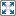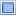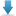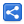# Python - Tuples

[Last Updated: Mar 9, 2022]

Python tuple is immutable ordered collection which can have duplicates.

The difference between Python lists and tuples is that, lists are mutable (can be changed), whereas, tuples are immutable (cannot be changed).

### Declaring tuples

```even_numbers = (2, 4, 6)
print(even_numbers)```
`(2, 4, 6)`

### Declaring a tuple of mixed types

```items = (2, "three", 2.3)
print(items)```
`(2, 'three', 2.3)`

### Using type() on a tuple

```even_numbers = (2, 4, 6)
print(type(even_numbers))```
`<class 'tuple'>`

### Accessing Tuple elements

Tuples can be accessed via zero-based indexing:

```even_numbers = (2, 4, 6)
print(even_numbers)
print(even_numbers)
print(even_numbers)```
`246`

### Accessing tuples from right side

```even_numbers = (2, 4, 6)
print(even_numbers[-1])
print(even_numbers[-2])
print(even_numbers[-3])```
`642`

### Finding index of an element

```alphas = ('a', 'b', 'c', 'd', 'e')
i = alphas.index('c')
print(i)```
`2`

### Finding index in sub-tuple

```alphas = ('a', 'b', 'a', 'c', 'a')
i = alphas.index('a', 2) # from index 2
print(i)
i = alphas.index('a', 3, 5) # from index 3 to index 5
print(i)```
`24`

### ValueError

ValueError is raised if element does not exist when we use index() method:

```alphas = ('a', 'b', 'c', 'd', 'e')
i = alphas.index('z')
print(i)```
`Traceback (most recent call last):     i = alphas.index('z')ValueError: tuple.index(x): x not in tuple`

### Getting length of a tuple

```even_numbers = (2, 4, 6)
length = len(even_numbers)
print(length)```
`3`

### Trying to replace an element

Since tuples are immutable data structures, an error is thrown if we try to replace elements:

```even_numbers = (2, 4, 6)
even_numbers = 10
print(even_numbers)```
`Traceback (most recent call last):     even_numbers = 10TypeError: 'tuple' object does not support item assignment`

Tuples also do not support appending/inserting new elements, deleting elements by values or by index, clearing all elements (via clear()), sorting (via sort()), reversing order (via revers()) and copy (via copy()).

### Removing whole tuple (removing from memory)

```alphas = ('a', 'b', 'c', 'd', 'e')
#removing complete tuple (just like other objects via del)
del alphas
print(alphas)```
`Traceback (most recent call last):     print(alphas)NameError: name 'alphas' is not defined`

### Index error

If we try to access a tuple outside of it's index range:

```even_numbers = (2, 4, 6)
even_numbers=8
print(even_numbers)```
`Traceback (most recent call last):     even_numbers=8TypeError: 'tuple' object does not support item assignment`

### Looping tuple

```even_numbers = (2, 4, 6)
for x in even_numbers:
print(x)```
`246`

### Looping by index

```even_numbers = (2, 4, 6)
length = len(even_numbers)
for x in range(length):
print(even_numbers[x])```
`246`

### Checking if an element exits in a tuple

```alphas = ('a', 'b', 'c', 'd', 'e')
result = 'c' in alphas
print(result)```
`True`

### Finding frequency of an element

```alphas = ('a', 'b', 'a', 'd', 'a')
i = alphas.count('a')
print(i)
# if element does not exist
i = alphas.count('z')
print(i)```
`30`

### Finding substrings

```alphas = ('a', 'b', 'c', 'd', 'e')
print(alphas[1:3])
print(alphas[:2])
print(alphas[2:])
```
`('b', 'c')('a', 'b')('c', 'd', 'e')`

## Example Project

Dependencies and Technologies Used:

• Python 3.10.2
 Python - Tuple ExamplesSelect AllDownload• python-tuple-examples
• scripts
• 01_declaring_tuples.py

 Share